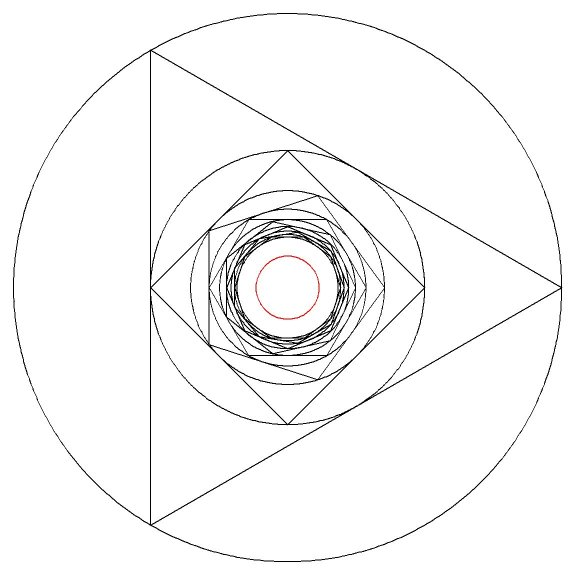# »  Solutions to puzzles in my National Review Online Diary

##August 2004

—————————

In my August diary I posed the following brain-teaser.

Draw a circle of radius 1 unit (foot, meter, mile, whatever). Inscribe an equilateral triangle in the circle, the three corners all on the circle's circumference. Now inscribe a smaller circle in the triangle, its circumference just touching the sides of the triangle at their mid-points.

Starting with that smaller inner circle, repeat the process; but this time inscribe a square instead of a triangle. Then inscribe a yet smaller circle in the square, its circumference just touching the sides of the square at their mid-points.

Starting with this yet smaller circle, inscribe a regular pentagon in it … and then a circle inside the pentagon, touching its sides. Keep going in this fashion, with a hexagon, a heptagon, and so on.

The circle you inscribe inside an N-gon will be the (N − 1)-th circle you've drawn. (The circle inscribed in the square, for example, is the third one you've drawn.) The radii of all the circles you have drawn up to that point will form a sequence of (N − 1) numbers, each smaller than the one before: 1, r2, r3, r4, … rN − 1. What is the limit of this sequence?

—————————

SolutionFor the solution, first note the following thing, which you can get from the merest exercise in elementary trigonometry.

Draw a regular N-gon (that is, a regular polygon with N sides).

Draw the circumscribing circle (i.e. the one that goes through all the vertices of the N-gon). Call its radius R.

Draw the inscribing circle (i.e. the one that touches all the sides of the N-gon). Call its radius r.

Then  r = R cos(π / N)

(If you prefer to measure angles in degrees rather than radians, just replace the "π" by "180.")

It follows from this that the circle inscribing the N-gon in my puzzle has radius

cos(π / 3) × cos(π / 4) × cos(π / 5) × … × cos(π / N)

So the question is: What happens to this quantity as N gets indefinitely large ("goes to infinity")?

Since the angles get closer and closer to zero, whose cosine is 1, there is a decent chance that this infinite product will converge to some number bigger than zero. In fact, brute computation shows that it does indeed converge to 0.1149420448 … I have shown this "limit circle" in red in the diagram.

But what is this number? Can we get a closed form for it?

I don't know the answer. So far as I am aware, it is known only as "the polygon-inscribing constant," and is unrelated to any other mathematical constants. Not that you can't get alternative expressions for it; but they are all worse than the one you started with. Take its log, for example, and apply the series for −log(cos(z)) in Abramowitz and Stegun's Handbook of Mathematical Functions:

−log(cos(z)) = z 2/2 + z 4/12 + z 6/45 + 17z 8/2520 + 31z 10/14175 + …

If you apply that to the successive angles and add up the results, you get an infinite series involving — yes! — zeta functions. It doesn't resolve to anything simple, though. Those coefficients are derived from the Bernoulli numbers; and ζ(N), for even values of N, resolves to an expression in Bernoulli numbers and powers of π; so you end up with a mess of Bernoulli numbers and powers of π.

A very good rational approximation (good to six decimal places) to the polygon-inscribing constant is 10/87.

Incidentally, you can also proceed in the opposite direction: That is, start with a circle of radius 1, circumscribe an equilateral triangle whose sides touch the circle, draw the circumcircle of that triangle, circumscribe a square, and so on. The radius of the limiting circle in that case is just the reciprocal of "the polygon-inscribing constant" — about 8.7000366252. For an interesting note on this, see the article "Mathematics and Modern Technology" in the August-September 1996 issue of American Mathematical Monthly.